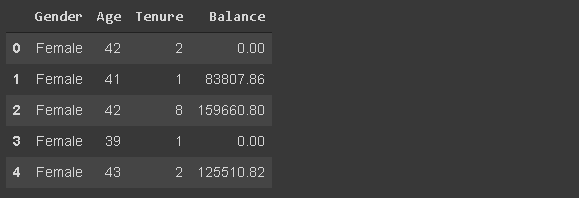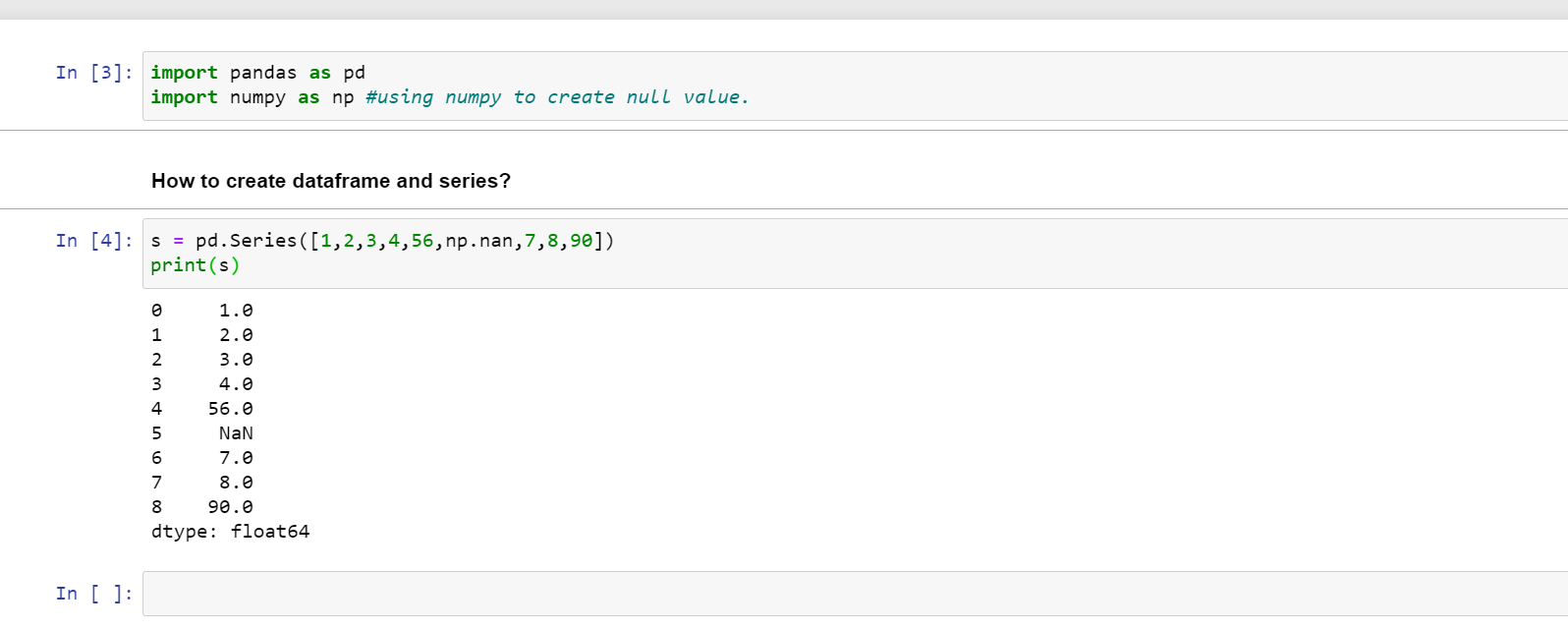1604502900

# 30 Examples to Master Pandas

Pandas is a widely-used data analysis and manipulation library for Python. It provides numerous functions and methods that expedite the data analysis and preprocessing steps.

Due to its popularity, there are lots of articles and tutorials about Pandas. This one will be one of them but heavily focusing on the practical side. I will do examples on a  customer churn dataset that is available on Kaggle.

The examples will cover almost all the functions and methods you are likely to use in a typical data analysis process.

Let’s start by reading the csv file into a pandas dataframe.

``````import numpy as np
import pandas as pd

df.shape
(1000,14)
df.columns
Index(['RowNumber', 'CustomerId', 'Surname', 'CreditScore', 'Geography', 'Gender', 'Age', 'Tenure', 'Balance', 'NumOfProducts', 'HasCrCard','IsActiveMember','EstimatedSalary', 'Exited'], dtype='object')
``````

### 1. Dropping columns

The drop function is used to drop columns and rows. We pass the labels of rows or columns to be dropped.

``````df.drop(['RowNumber', 'CustomerId', 'Surname', 'CreditScore'], axis=1, inplace=True)

df.shape
(10000,10)
``````

The axis parameter is set as 1 to drop columns and 0 for rows. The inplace parameter is set as True to save the changes. We dropped 4 columns so the number of columns reduced to 10 from 14.

### 2. Select particular columns while reading

We can read only some of the columns from the csv file. The list of columns is passed to the usecols parameter while reading. It is better than dropping later on if you know the column names beforehand.

``````df_spec = pd.read_csv("/content/churn.csv", usecols=['Gender', 'Age', 'Tenure', 'Balance'])

``````(image by author)

#artificial-intelligence #data-science #programming #machine-learning #data-analysis

## Buddha Community1586702221

## Python Pandas Objects - Pandas Series and Pandas Dataframe

In this post, we will learn about pandas’ data structures/objects. Pandas provide two type of data structures:-

### Pandas Series

Pandas Series is a one dimensional indexed data, which can hold datatypes like integer, string, boolean, float, python object etc. A Pandas Series can hold only one data type at a time. The axis label of the data is called the index of the series. The labels need not to be unique but must be a hashable type. The index of the series can be integer, string and even time-series data. In general, Pandas Series is nothing but a column of an excel sheet with row index being the index of the series.

### Pandas Dataframe

Pandas dataframe is a primary data structure of pandas. Pandas dataframe is a two-dimensional size mutable array with both flexible row indices and flexible column names. In general, it is just like an excel sheet or SQL table. It can also be seen as a python’s dict-like container for series objects.

#python #python-pandas #pandas-dataframe #pandas-series #pandas-tutorial1602550800

## Pandas in Python

Pandas is used for data manipulation, analysis and cleaning.

What are Data Frames and Series?

Dataframe is a two dimensional, size mutable, potentially heterogeneous tabular data.

It contains rows and columns, arithmetic operations can be applied on both rows and columns.

Series is a one dimensional label array capable of holding data of any type. It can be integer, float, string, python objects etc. Panda series is nothing but a column in an excel sheet.

### How to create dataframe and series?

s = pd.Series([1,2,3,4,56,np.nan,7,8,90])

print(s)How to create a dataframe by passing a numpy array?

1. d= pd.date_range(‘20200809’,periods=15)
2. print(d)
3. df = pd.DataFrame(np.random.randn(15,4), index= d, columns = [‘A’,’B’,’C’,’D’])
4. print(df)

#pandas-series #pandas #pandas-in-python #pandas-dataframe #python1616050935

## WORKING WITH GROUPBY IN PANDAS

In my last post, I mentioned the groupby technique  in Pandas library. After creating a groupby object, it is limited to make calculations on grouped data using groupby’s own functions. For example, in the last lesson, we were able to use a few functions such as mean or sum on the object we created with groupby. But with the aggregate () method, we can use both the functions we have written and the methods used with groupby. I will show how to work with groupby in this post.

#pandas-groupby #python-pandas #pandas #data-preprocessing #pandas-tutorial1623897480

## Practice Problems: How To Use Pandas DataFrames' GroupBy Method

It’s now time for some practice problems! See below for details on how to proceed.

### Course Repository & Practice Problems

All of the code for this course’s practice problems can be found in this GitHub repository.

There are two options that you can use to complete the practice problems:

• Open them in your browser with a platform called Binder using this link (recommended)
• Download the repository to your local computer and open them in a Jupyter Notebook using Anaconda (a bit more tedious)

Note that binder can take up to a minute to load the repository, so please be patient.

Within that repository, there is a folder called `starter-files` and a folder called `finished-files`. You should open the appropriate practice problems within the `starter-files` folder and only consult the corresponding file in the `finished-files` folder if you get stuck.

The repository is public, which means that you can suggest changes using a pull request later in this course if you’d like.

#pandas #groupby methods #pandas dataframe #example #practice problems: how to use pandas dataframes' groupby method #practice problems1616395265

## Reading and Writing Data in Pandas

In my last post, I mentioned summarizing and computing descriptive statistics  using the Pandas library. To work with data in Pandas, it is necessary to load the data set first. Reading the data set is one of the important stages of data analysis. In this post, I will talk about reading and writing data.

Before starting the topic, our Medium page includes posts on data science, artificial intelligence, machine learning, and deep learning. Please don’t forget to follow us on Medium 🌱 to see these posts and the latest posts.

Let’s get started.